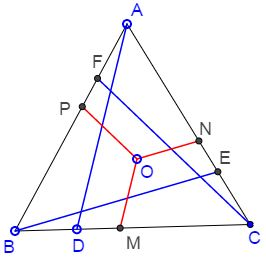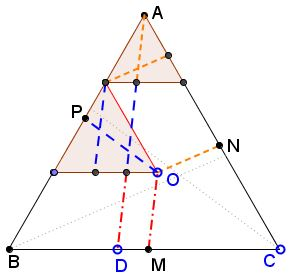# Slanted Viviani, PWW

### Problem

$ABC$ is an equilateral triangle. Cevians $AD,$ $BE,$ $CF$ are equal (and, for the esthetics' sake, equally inclined to the corresponding sides.) Points $M,N,P$ are on $BC,AC,AB,$ respectively, such that $OM\parallel AD,$ $ON\parallel BE,$ and $OP\parallel CF.$Prove that $OM+ON+OP=AD.$

### Proof

The proof is supposed to be self-explanatory.In case of difficulties in interpretation, please, have a look at a more explicit variant.

### Acknowledgment

The illustration is by Grégoire Nicollier.

• Equilateral and 3-4-5 Triangles
• Rusty Compass Construction of Equilateral Triangle
• Equilateral Triangle on Parallel Lines
• Equilateral Triangle on Parallel Lines II
• When a Triangle is Equilateral?
• Viviani's Theorem
• Viviani's Theorem (PWW)
• Tony Foster's Proof of Viviani's Theorem
• Viviani in Isosceles Triangle
• Viviani by Vectors
• Slanted Viviani
• Morley's Miracle
• Triangle Classification
• Napoleon's Theorem
• Sum of Squares in Equilateral Triangle
• A Property of Equiangular Polygons
• Fixed Point in Isosceles and Equilateral Triangles
• Parallels through the Vertices of Equilateral Triangle
•